Question

# A volume of 90.0 mL of a 0.750 M HNO3 solution is titrated with 0.730 M...

A volume of 90.0 mL of a 0.750 M HNO3 solution is titrated with 0.730 M KOH. Calculate the volume of KOH required to reach the equivalence point.

Express your answer to three significant figures and include the appropriate units.

We need at least 10 more requests to produce the answer.

0 / 10 have requested this problem solution

The more requests, the faster the answer.

All students who have requested the answer will be notified once they are available.

#### Earn Coins

Coins can be redeemed for fabulous gifts.

Similar Homework Help Questions
• ### A volume of 50.0 mL of a 0.690 M HNO3 solution is titrated with O.230 M...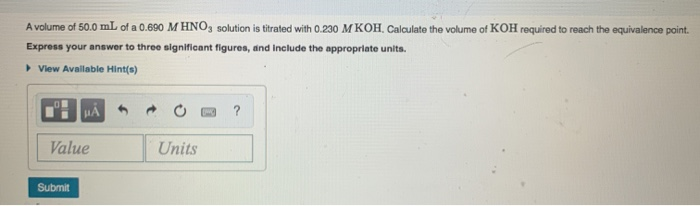A volume of 50.0 mL of a 0.690 M HNO3 solution is titrated with O.230 M KOH, Calculate the volume of KOH required to reach the equivalence point. Express your answer to three significant figures, and include the appropriate units. View Available Hint(s) ? Value Units Submit

• ### Part A volume of 40.0 mL of a 0.820 M HNO3 solution is titrated with 0.390...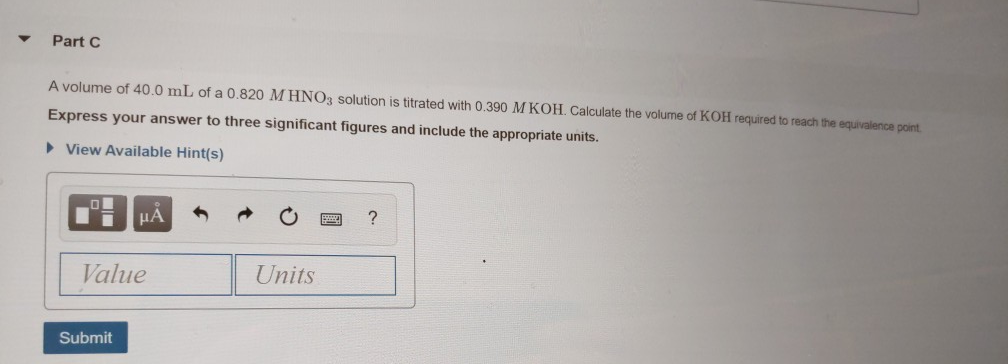Part A volume of 40.0 mL of a 0.820 M HNO3 solution is titrated with 0.390 M KOH. Calculate the volume of KOH required to reach the equivalence point Express your answer to three significant figures and include the appropriate units. ► View Available Hint(s) Value Units Submit

• ### Part C A volume of 40.0 mL of a 0.280 M HNO3 solution is titrated with...

Part C A volume of 40.0 mL of a 0.280 M HNO3 solution is titrated with 0.820 M KOH. Calculate the volume of KOH required to reach the equivalence point. Express your answer to three significant figures, and include the appropriate units. How many moles of Ba(OH)2 are present in 235 mL of 0.800 M Ba(OH)2? Express your answer with the appropriate units.

• ### Part A volume of 30,0 mL of a 0.470 MHNO, solution is titrated with 0.800 M...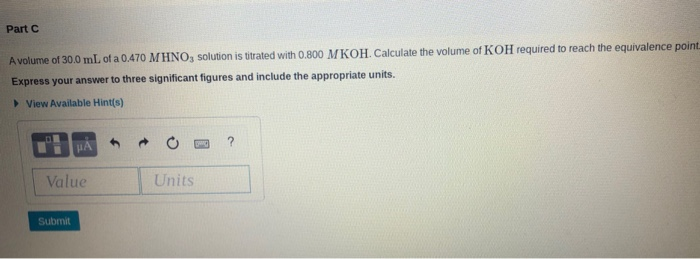Part A volume of 30,0 mL of a 0.470 MHNO, solution is titrated with 0.800 M KOH. Calculate the volume of KOH required to reach the equivalence point Express your answer to three significant figures and include the appropriate units. View Available Hint(s) Value Units Submit

• ### A volume of 100 mL of a 0.440 M HNO; solution is titrated with 0.760 M...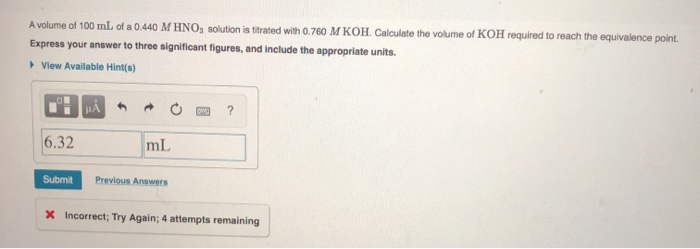A volume of 100 mL of a 0.440 M HNO; solution is titrated with 0.760 M KOH. Calculate the volume of KOH required to reach the equivalence point. Express your answer to three significant figures, and include the appropriate units. View Available Hint(s) H ? 6.32 mL Submit Previous Answers X Incorrect; Try Again; 4 attempts remaining

• ### A volume of 91.5 mL of a 0.205 M HNO3 solution is titrated with 0.196 M...

A volume of 91.5 mL of a 0.205 M HNO3 solution is titrated with 0.196 M KOH. Calculate the volume of KOH required to reach the equivalence point. Enter in units of ml and to 2 decimal places.

• ### A volume of 60.0 mL of a 0.900 M HNO3 solution is titrated with 0.820 M...

A volume of 60.0 mL of a 0.900 M HNO3 solution is titrated with 0.820 M KOH Calculate the volume of KOH required to reach the equivalence point.

• ### Animation-Acid-Base Titration C5o Part A volume of 60.0 mL of 0.120 M HNO, solution is titrated with 0.740 MKOH Cal...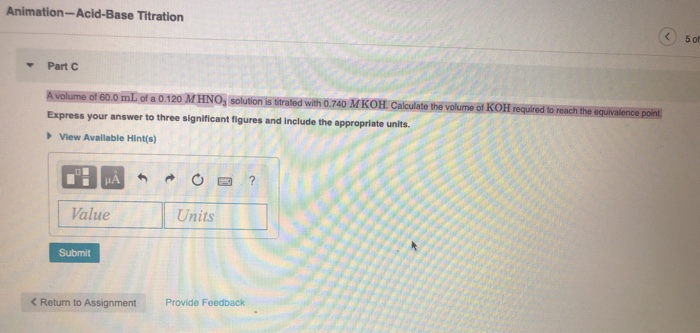Animation-Acid-Base Titration C5o Part A volume of 60.0 mL of 0.120 M HNO, solution is titrated with 0.740 MKOH Calculate the volume of KOH required to reach the equivalence point Express your answer to three significant figures and include the appropriate units. View Available Hint(s) HAR O Value Submit < Return to Assignment Provide Feedback

• ### A volume of 80.0 mL of a 0.790 M HNO Calculate the volume of KOH required...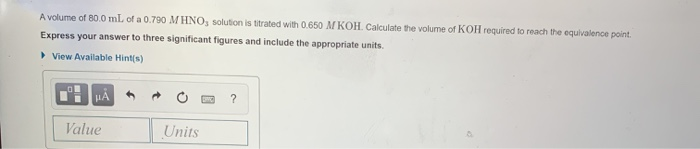A volume of 80.0 mL of a 0.790 M HNO Calculate the volume of KOH required to reach the equlvalence point. solution is titrated with 0.650 M KOH Express your answer to three significant figures and include the appropriate units. View Available Hint(s) Value Units

• ### 1)Use the Henderson–Hasselbalch equation to calculate the pH of: a solution that is 13.5 g of...

1)Use the Henderson–Hasselbalch equation to calculate the pH of: a solution that is 13.5 g of HF and 25.0 g of NaF in 125 mL of solution Express your answer using two decimal places. 2) A volume of 20.0 mL of a 0.380 M HNO3 solution is titrated with 0.850 M KOH. Calculate the volume of KOH required to reach the equivalence point. Express your answer to three significant figures and include the appropriate units.